## Continuous Function

A continuous function is a Function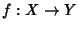where the pre-image of every Open Set in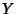is Open in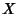. A function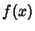in a single variableis said to be continuous at point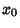if

1.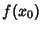is defined, so thatis in the Domain of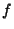.

2.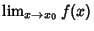exists forin the Domain of.

3.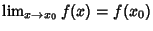,

where lim denotes a Limit. Ifis Differentiable at point, then it is also continuous at. Ifand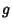are continuous at, then

1.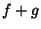is continuous at.

2.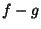is continuous at.

3.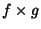is continuous at.

4.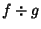is continuous atif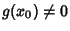and is discontinuous atif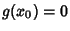.

5.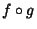is continuous at, wheredenotes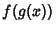, the Composition of the functionsand.